## General problem-solving tips

### Quick description

Many Tricki articles are about methods for solving particular classes of problems. But when one is doing mathematical research, many of the most useful tips are not methods of this kind but are more like general research strategies that can in principle be applied to virtually any mathematical problem. The Tricki contains descriptions of several of these, and they are listed below.

If you would like to write an article in this category, try to adhere to the Tricki format: your article will be much more useful if you can give examples of mathematical situations where your advice is helfpul. The following dead links and descriptions of articles that have yet to be written give some idea of what we mean by a general research strategy.

### The articles

Don't start from scratch Quick description ( A common mistake people make when trying to answer a mathematical question is to work from first principles: it is almost always easier to modify something you already know. This article illustrates the point with examples that range from simple arithmetic to problems from the forefront of research. )

Hunt for analogies Quick description ( It is surprising how often the following general approach to problem-solving is successful: you have a problem you don't yet know how to solve; you think of a somewhat similar context where you can formulate an analogous problem that you do know how to solve; you then work out what the corresponding solution ought to be in the context you started with. Even quite loose analogies can do a wonderful job of guiding you in the right direction. )

Mathematicians need to be metamathematicians Quick description ( If you want to prove a theorem, then one way of looking at your task is to regard it as a search, amongst the huge space of potential arguments, for one that will actually work. One can often considerably narrow down this formidable search by thinking hard about properties that a successful argument would have to have. In other words, it is a good idea to focus not just on the mathematical ideas associated with your hoped-for theorem, but also on the properties of different kinds of proofs. This very important principle is best illustrated with some examples. )

Think about the converse Quick description ( If you are trying to prove a mathematical statement, it is often a good idea to think about its converse, especially if the converse is not obvious. This is particularly useful if the statement you are trying to prove is a lemma that you would like to use to prove something else. Some examples will help to explain why. More obviously, it is useful if you are trying to prove a result that would, if true, be best possible. )

Try to prove the opposite Quick description ( If you want to prove a mathematical statement, try proving the negation of that statement. Very often it gives you an insight into why the original statement is true, and sometimes you discover that it is not true. This tip can be iterated. )

Look for related problems Quick description ( When you are trying to solve a problem, it can be very helpful to formulate similar-looking problems and think about those too. Sometimes they turn out to be interesting in themselves, and sometimes they lead you to ideas that are useful for the original problem. )

Work on clusters of problems Quick description ( It is not usually a good research strategy to think about one isolated problem, unless you are already some way to solving it. Solving a problem involves a certain degree of luck, so your chances of success are much greater if you look at a cluster of related problems. )

Look at small cases Quick description ( Can't see how to solve a problem? Then see if you can solve it in special cases. Some special cases will be too easy to give you a good idea of how to approach the main problem, and some will be more or less as difficult as the main problem, but if you search for the boundary between these two extremes, you will often discover where the true difficulty lies and what it is. And that is progress. )

Try to prove a stronger result Quick description ( As a student, one is asked to prove many statements that have been carefully designed so that their hypotheses are exactly the appropriate ones for deducing the conclusion. This makes it possible to design "trick" questions with unnecessarily strong hypotheses: these questions can be hard if one tries to use the hypotheses as they stand, since their full strength is irrelevant. The problems that arise when one is doing research are often of the "trick" variety: one has not been set them by a benign professor in the sky. So it is a good idea to investigate whether weaker hypotheses will suffice. As with looking at small cases, this can help one to locate the true point of difficulty of a problem. Similarly, if you are trying to find an example of a mathematical structure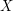that has a certain property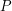, it may be easier to look for anthat has a stronger property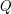. And even if you fail, you are likely to understand much better what is required to find anthat satisfies. )

Prove a consequence first Quick description ( This is the flip side of "Try to prove a stronger result"; if one wants to prove some statement, it can be useful to first prove a weaker consequence of that statement, and then use that weaker result as a stepping stone to the full result.)

Think axiomatically even about concrete objects Quick description ( If you are trying to prove a fact about the exponential function, it may be easier not to use any of the common definitions of this function, but to use instead a few properties that it has, of which the most important is that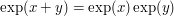. In general, it is often possible to turn concrete problems into abstract ones in this way, and doing so can considerably clarify the problems and their solutions. )

Temporarily suspend rigor Quick description ( The final proof of a result should of course be fully rigorous. But this certainly does not prevent one from suspending rigor in order to locate the right proof strategy to pursue. For instance, if one needs to compute some complicated integral expression, one can temporarily suspend concerns about whether operations such as interchange of integrals is actually justified, and go ahead and perform these operations anyway in order to find a plausible answer. One can always go back later and try to make the argument more rigorous.)

Turn off all but one of the difficulties Quick description ( A problem may have several independent difficulties plaguing it; for instance one may need to establish an estimate which is uniform both with respect to a large parameter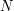, and an independent small parameter. In that case, one can often proceed by passing to a special case in which only one of the difficulties is "active", solving each of these basic special cases, and then try to merge the arguments together. For instance, one could set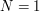and get an argument which is uniform as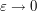, then set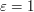and get an argument which is uniform as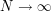, then try to put them together.)

Simplify your problem by generalizing it Quick description ( Sometimes if you generalize a statement, the result is easier to prove. There are several reasons for this, discussed in separate articles linked to from this page. )

If you don't know how to make a decision, then don't make it Quick description ( Very often a proof requires one to choose some object that will make the rest of the proof work. And very often it is far from obvious how to make the choice. Often a good way of getting round the problem is to take an arbitrary object of the given type, give it a name, and continue with the proof as if you had chosen. Along the way, you will find that you need to assume certain properties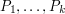of. Then your original problem is reduced to the question "Does there exist an object of the given type with properties?" Often, this is a much more straightforward question than the main problem you were trying to solve. )

If an argument looks promising but needs some technical hypothesis, try assuming that hypothesis for now, but aim to remove it later Quick description ( If you have an idea on how to solve a problem, but it requires a hypothesis that one does not actually have, one doesn't necessarily have to abandon the strategy entirely; one may try instead for a conditional result assuming the hypothesis instead. If that is successful, one can then try to look for ways to remove the extra hypothesis. )